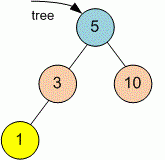Competitions

# Tree Maximum depth

Given a binary tree, find its maximum depth. The maximum depth is the number of nodes along the longest path from the root node down to the farthest leaf node.

Definition of a tree:

// Java
class TreeNode
{
public:
int val;
TreeNode left;
TreeNode right;
TreeNode(int x) {
val = x;
left = NULL;
right = NULL;
};

// C++
class TreeNode
{
public:
int val;
TreeNode *left;
TreeNode *right;
TreeNode(int x) : val(x), left(NULL), right(NULL) {}
};


Implement function maxDepth that returns the maximum depth of the tree.

// Java
int maxDepth(TreeNode tree)

// C++
int maxDepth(TreeNode *tree)


#### ExampleFunction maxDepth returns 3 because the longest path from the root 5 to the farthest leaf 1 contains 3 nodes.

Time limit 1 second
Memory limit 128 MiB
Author Mykhailo Medvediev